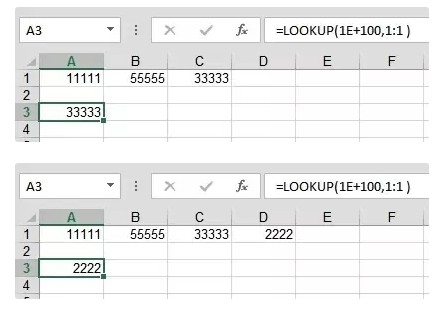# Some point in the past was the way to reference the last value on a row or on a column

Jan 12, 2020 • edited Jan 13, 2020I don't know how “cool” it is, but the most useful thing I have come across at some point in the past was the way to reference the last value on a row or on a column.

If you want to reference the last value on row 24:
=LOOKUP(1E100, 24:24)

And if you want to reference the last value on column G:
=LOOKUP(1E100, G:G)

And if you're wondering the same thing I was when I discovered this formula…

1E100 is a scientific notation for a very large number, meaning 1 followed by 100 zeros. There are many notations you can use for “very large number” (for example 9.999999999E+307, which is the largest number Excel recognizes), but 1E100 is much easier to remember and to type.

And the explanation for this trick is that LOOKUP searches for the last number in a range that is smaller than or equal to the lookup value, so when it looks for that big number and doesn't find it, it returns the last value it comes across.

Here's also a visual example, for anyone who learns better through examples.Notice the formula staying the same, but the result still referencing the new last value that is now in a different cell.

Note: LOOKUP doesn't work with text, and this only works as long as you don't have any values in your data that exceed 1E100.

#Tips#Rows#Columns

9 helpful tips and formulas in EXCEL

20 Excel Tricks for Beginners & Experts